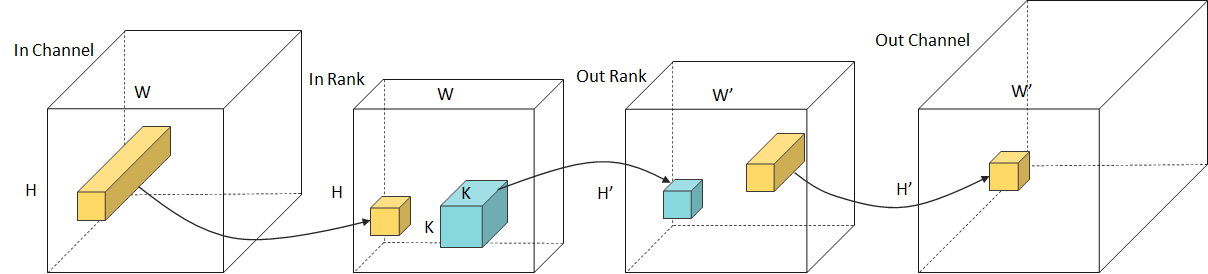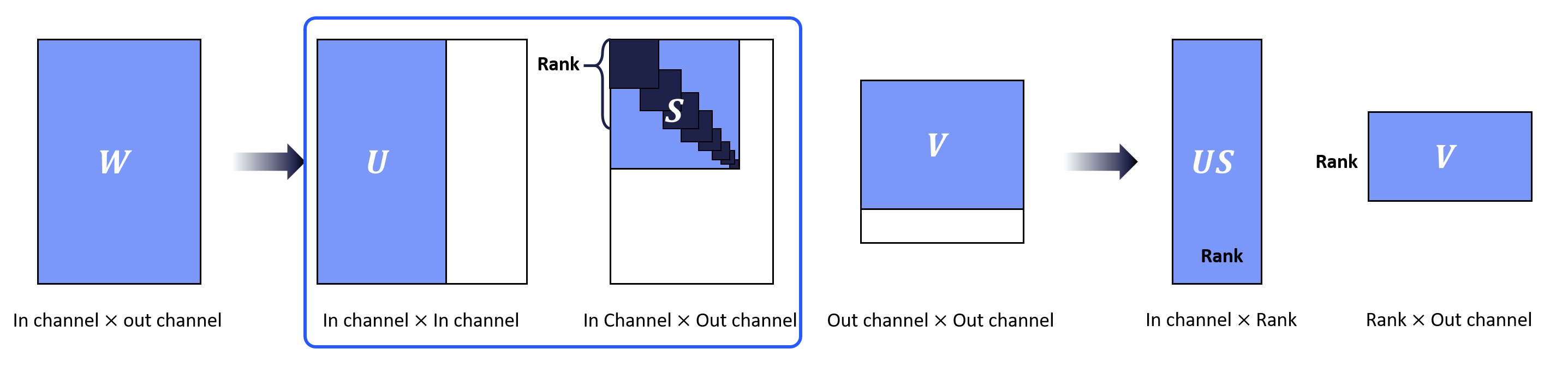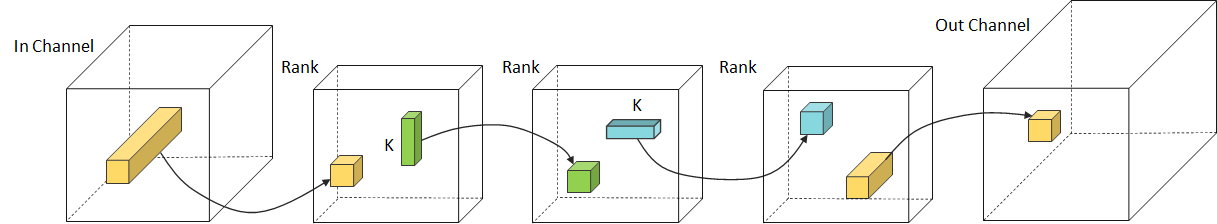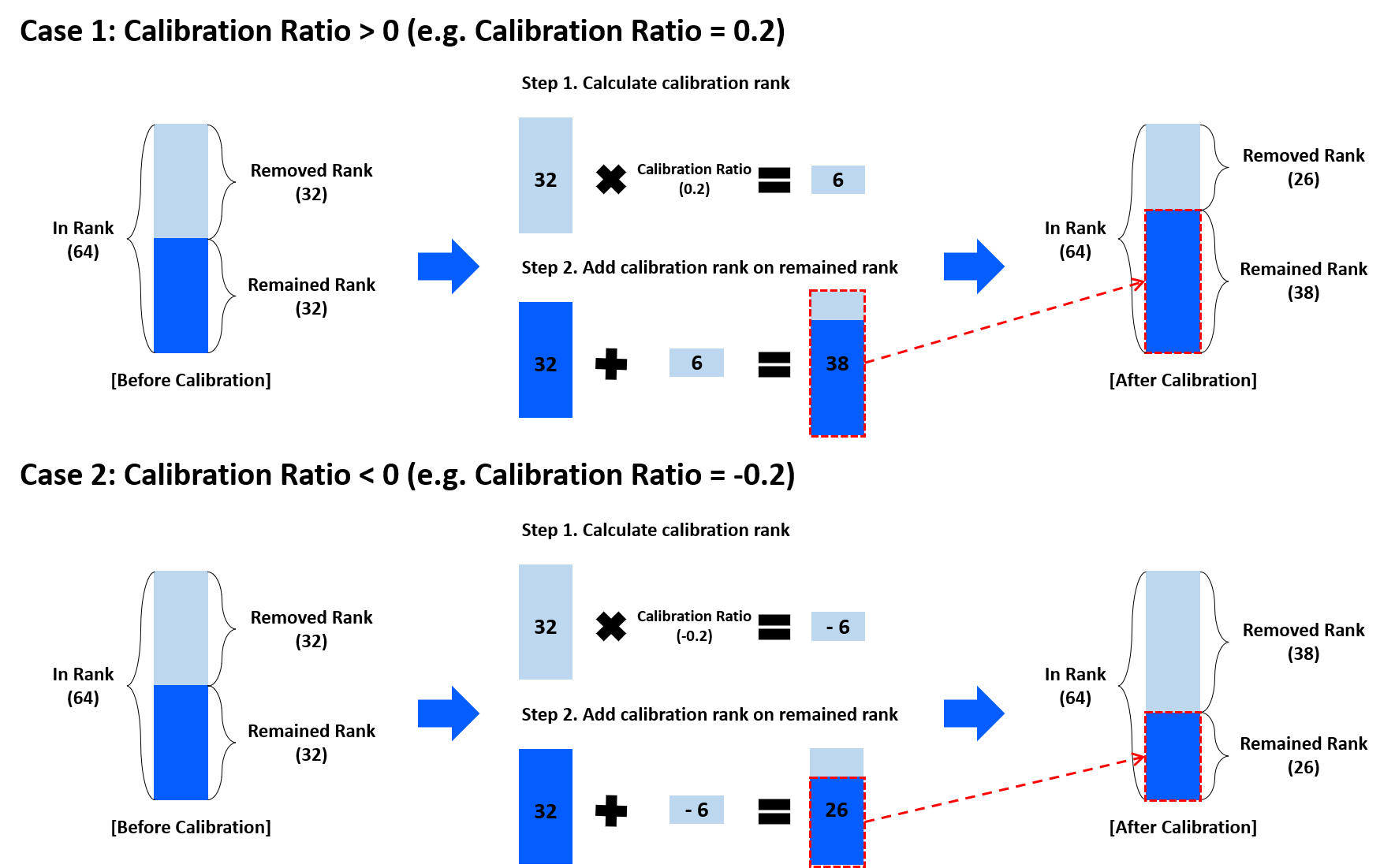# Method: Filter Decomposition

## Model Compression

The goal of model compression is to achieve a model that is simplified from the original without performance deterioration. By compressing the large model, the user can reduce the storage and computational cost and allow to use in real-time applications.

NetsPresso supports the following compression methods.

• Structured Pruning
• Filter Decomposition

## What is "Filter Decomposition"?

Filter decomposition is to approximate original weights into lightweight representations via low-rank approximations to reduce the computational cost.

## Supported method

Model Compressor supports the three following methods, Tucker Decomposition, Singular Value Decomposition (SVD), and Canonical Polyadic (CP) Decomposition.

1) Tucker Decomposition
Tucker decomposition decomposes the convolution with a 4D kernel tensor into two factor matrices and one small core tensor.• In Channel : The number of input channel in each layer.
• Out Channel : The number of output channel in each layer.
• In Rank : The number of input channel of core tensor that represent relation level of low-rank factor matrix.
• Out Rank : The number of output channel of core tensor that represent relation level of low-rank factor matrix.

2) Singular Value Decomposition (SVD)

Singular Value Decomposition (SVD) decomposes the pointwise convolution or fully-connected layer into two pointwise or fully-connected layers.• In Channel: The number of input channels of the target layer
• Out Channel: The number of output channels of the target layer
• Rank: The condition number of weight matrix W

CP Decomposition replaces an original tensor with a linear combination of rank one tensors.• In Channel : The number of input channel in each layer.
• Out Channel : The number of output channel in each layer.
• Rank : A sum of N-way outer products of rank-one tensor for estimating original convolution filter.

If you want to know more about filter decomposition methods:

## "Recommendation" in Model Compressor

The "Recommendation" allows the user to set proper compress hyperparameter of the filter decomposition, in-rank, and out-rank.

• "Recommendation" calculates the ranks based on the Variational Bayesian Matrix Factorization (VBMF)
• Users can manipulate the compression amount by using the calibration ratio of the Recommendation.
• "Recommendation" is only available for Tucker Decomposition and Singular value decomposition methods. (CP Decomposition will be available soon.)

Detailed information of the Calibration ratio

• The default value of the calibration ratio is 0.0, which returns the same rank as VBMF
• The smaller the calibration ratio(>= -1, <=1), the smaller the model will be returned.
• Example of how the rank is decided by the calibration ratio.
•## What you can do with Model Compressor

• User can compress the model using one of the filter decomposition methods.
• After selecting the layer to be compressed, user fills in the rank value in the range. (available rank values are different in each layer)
• To use Tucker Decomposition, user have to input two values, in/out rank
• To use CP Decomposition or Singular Value Decomposition, only one value, rank is needed.
• If user is hard to input proper values, you might as well use recommendation function.
• 'Recommendation' function based on VBMF algorithm and user can control the compression level through 'calibration ratio' additionally.

🚧

Not Supported Layers

• Group convolutional layer is currently not supported and will be updated in the near future.